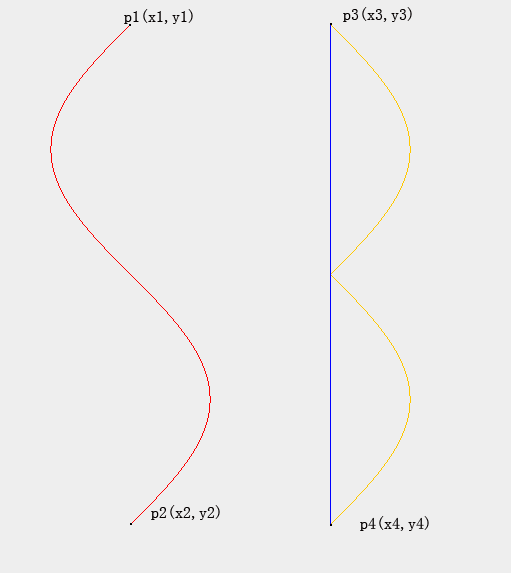很SB的Java程序在此for(int i = 0 ; points.length ; i++){

robot.delay(speed);

robot.mouseMove(points[i].x， points[i].y);

}


final int m = 150;// 上下的边距
final int xpoints[];// 保存x坐标.因为y是递增的，规律简单，可以不用保存
final int dist = 200;// 两条曲线间的距离
final int y1 = m,
y2 = Toolkit.getDefaultToolkit().getScreenSize().height - m;
final int d = y2 - y1;
final double n = (y2 - y1) / (2 * Math.PI);
final int x1 = (Toolkit.getDefaultToolkit().getScreenSize().width - dist)
/ 2 - (int) Math.round(n);
final int x3 = x1 + dist;
xpoints = new int[d * 3];// 点的总量是d*3;
double i = -Math.PI;
int nn = 0;
while (nn < d) {
xpoints[nn] = (int) Math.round(x1 + n * Math.sin(i));// 红色轨迹
xpoints[d + nn] = x3;// 蓝色轨迹
xpoints[2 * d + nn] = x3 + Math.abs(xpoints[nn] - x1);// 橙色轨迹
nn++;
i += 1 / n;
}


final int speed=5;//休眠时间，就是是鼠标运动的速度
try {
Robot r = new Robot();//实例化一个Robot对象
int tmpY= 0 ;
while(true){
if(nn>=d&&nn<=2*d)
//为保持鼠标移动速度的一致，直线的距离要比两条曲线的距离短，而实际的关键点是一样多.所以每个关键像素的
//停留时间都要缩短一下，当然1/2是不准确的估计.见@http://dourok.info/2010/08/316/最上面的红色公式.
r.delay(speed/2);
else
r.delay(speed);
r.mouseMove(xpoints[nn]，(tmpY+y1));//mouseMove移动的是绝对位置而不是相对位置
tmpY=(tmpY+1)%(d);
nn=(nn+1)%xpoints.length;//让鼠标不断循环的画轨迹
}
}catch (AWTException ex) {
ex.printStackTrace();
}


Robot的强大还在于它不只能控制鼠标，键盘的任意行为，还有

public BufferedImage createScreenCapture(Rectangle screenRect)这个方法用来获取屏幕在Rect区域内的图像。基本上有这个方法，做抓屏软件就不是什么问题了。

public Color getPixelColor(int x,int y)这个方法是返回给定屏幕坐标处的像素颜色。是不是感觉更按键精灵的功能很相像。

keyboard_arrow_up